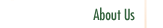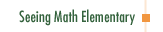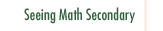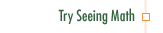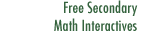Geometry: Calculating the Area of a Triangle

In Geometry: Calculating the Area of a Triangle, a group of fifth grade students builds a foundation for understanding the area of a triangle and finds methods to calculate it before learning the standard formula.

Participants in this course:

• Examine the difference between conceptual and procedural definitions.
• Explore how a conceptual definition of area informs methods to measure the area of a triangle.
• Examine questioning strategies that clarify concepts and support discussion.
• Explore how writing mathematics in journals builds mathematical understanding.
• Design innovative tools to assess understanding by using existing test activities or questions.
• Examine different methods of representation and how they affect strategies for problem solving.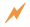Click to Get Seeing Math™ ElementaryCourse Listing Effective Questioning in the Mathematics Classroom Foundations of EffectiveMathematics Teaching Formative Assessment in the Mathematics Classroom Number & Operations: Division with Remainders Number & Operations: The Magnitude of Fractions Number & Operations: Broken Calculator Geometry: 2D and 3D Figures Geometry: Calculating Area of a Triangle Data Analysis and Probability: Using Data to Make Predictions Data Analysis and Probability: Measures of Center Pre-Algebra: Pan Balance Equations Pre-Algebra: Patterns and Functions